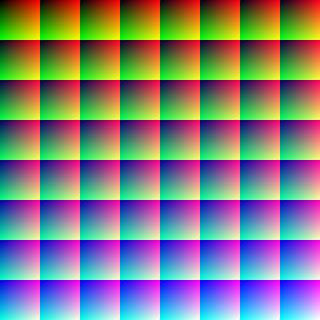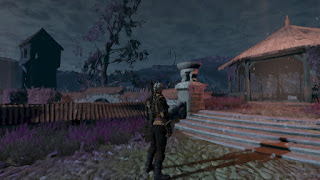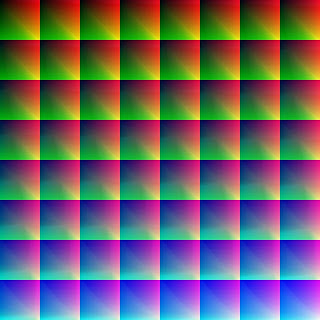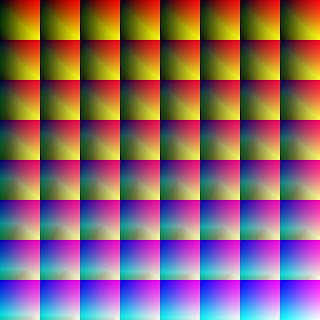## środa, 11 marca 2020

### Reverse engineering the rendering of The Witcher 3, part 18 - color grading

This post is a part of the series "Reverse engineering the rendering of The Witcher 3".

One of the postfx effects you can encounter pretty much everywhere in The Witcher 3 is color grading (aka color correction). The idea is to use a lookup table (LUT) texture to map one color set to another.

A usual workflow looks like this: there is a neutral (output color = input color) lookup table, which is edited in tools like Adobe Photoshop - enhancing contrast/brightness/saturation/hue etc... all sorts of modifications and adjustments which could be quite expensive to calculate in real-time. Thanks to LUTs, they can be replaced with cheaper texture lookups.

There are at least 3 different kinds of color LUT tables I'm aware of: 3D ones, "long" 2D ones and "square" 2D ones.A neutral "long" 2D LUTA neutral "square" 2D LUT

Nice OpenGL implementation with online demo
Color grading with Look-up Textures (LUT)
GPU Gems 2 article - color grading with 3D textures
UE4 docs about creating and using color LUTs

Let's take a look at the example LUT which is used in White Orchard, near the beginning of the game - most of green was changed to yellow:

The Witcher 3 uses 512x512 2D lookup textures.
As a general rule, color grading is expected to work in LDR space. This brings 2563 possible input values - more than 16 million combinations which are going to be mapped to only 5122=262 144 values. To cover whole input range, bilinear sampling is used.

And now comparison screenshots: before and after color grading pass.

As you can see, the difference is subtle yet noticeable - sky has a bit more orangish tint.

As for The Witcher 3 implementation, both input and output rendertargets are fullscreen floating-point (R11G11B10) textures. Interestingly, in this particular scene the brightest input pixel channels (near the Sun) have values exceeding 1.0f - even up to ~2.0f!

Here is the pixel shader assembly:
`````` ps_5_0
dcl_globalFlags refactoringAllowed
dcl_constantbuffer cb3, immediateIndexed
dcl_sampler s0, mode_default
dcl_sampler s1, mode_default
dcl_resource_texture2d (float,float,float,float) t0
dcl_resource_texture2d (float,float,float,float) t1
dcl_input_ps linear v1.xy
dcl_output o0.xyzw
dcl_temps 5
0: max r0.xy, v1.xyxx, cb3.xyxx
1: min r0.xy, r0.xyxx, cb3.zwzz
2: sample_indexable(texture2d)(float,float,float,float) r0.xyzw, r0.xyxx, t0.xyzw, s0
3: log r1.xyz, abs(r0.xyzx)
4: mul r1.xyz, r1.xyzx, l(0.454545, 0.454545, 0.454545, 0.000000)
5: exp r1.xyz, r1.xyzx
6: mad r2.xyz, r1.xyzx, l(1.000000, 1.000000, 0.996094, 0.000000), l(0.000000, 0.000000, 0.015625, 0.000000)
7: min r2.xyz, r2.xyzx, l(1.000000, 1.000000, 1.000000, 0.000000)
8: min r2.z, r2.z, l(0.999990)
9: add r2.xy, r2.xyxx, l(0.007813, 0.007813, 0.000000, 0.000000)
10: mul r2.xyzw, r2.xyzz, l(0.996094, 0.996094, 64.000000, 8.000000)
11: max r2.xy, r2.xyxx, l(0.015625, 0.015625, 0.000000, 0.000000)
12: min r2.xy, r2.xyxx, l(0.984375, 0.984375, 0.000000, 0.000000)
13: round_ni r3.xz, r2.wwww
14: mad r2.z, -r3.x, l(8.000000), r2.z
15: round_ni r3.y, r2.z
16: mul r2.zw, r3.yyyz, l(0.000000, 0.000000, 0.125000, 0.125000)
17: mad r2.xy, r2.xyxx, l(0.125000, 0.125000, 0.000000, 0.000000), r2.zwzz
18: sample_l(texture2d)(float,float,float,float) r2.xyz, r2.xyxx, t1.xyzw, s1, l(0)
19: mul r2.w, r1.z, l(63.750000)
20: round_ni r2.w, r2.w
21: mul r1.w, r2.w, l(0.015625)
22: mad r1.z, r1.z, l(63.750000), -r2.w
23: min r1.xyw, r1.xyxw, l(1.000000, 1.000000, 0.000000, 1.000000)
24: min r1.w, r1.w, l(0.999990)
25: add r1.xy, r1.xyxx, l(0.007813, 0.007813, 0.000000, 0.000000)
26: mul r1.xy, r1.xyxx, l(0.996094, 0.996094, 0.000000, 0.000000)
27: max r1.xy, r1.xyxx, l(0.015625, 0.015625, 0.000000, 0.000000)
28: min r1.xy, r1.xyxx, l(0.984375, 0.984375, 0.000000, 0.000000)
29: mul r3.xy, r1.wwww, l(64.000000, 8.000000, 0.000000, 0.000000)
30: round_ni r4.xz, r3.yyyy
31: mad r1.w, -r4.x, l(8.000000), r3.x
32: round_ni r4.y, r1.w
33: mul r3.xy, r4.yzyy, l(0.125000, 0.125000, 0.000000, 0.000000)
34: mad r1.xy, r1.xyxx, l(0.125000, 0.125000, 0.000000, 0.000000), r3.xyxx
35: sample_l(texture2d)(float,float,float,float) r1.xyw, r1.xyxx, t1.xywz, s1, l(0)
37: mad r1.xyz, r1.zzzz, r2.xyzx, r1.xywx
38: log r1.xyz, abs(r1.xyzx)
39: mul r1.xyz, r1.xyzx, l(2.200000, 2.200000, 2.200000, 0.000000)
40: exp r1.xyz, r1.xyzx
41: mad r1.xyz, cb3.zzzz, r1.xyzx, -r0.xyzx
42: mad o0.xyz, cb3.yyyy, r1.xyzx, r0.xyzx
43: mov o0.w, r0.w
44: ret
``````

In general, The Witcher 3 doesn't reinvent the wheel here and uses a lot of "security" code. Makes sense since this is one of the effects when you have to be extra careful with texture coordinates.

Still two LUT fetches are needed as it's a consequence of using 2D texture - this is to simulate bilinear sampling for the blue channel. In the OpenGL implementation above merging of these two fetches is based on fractional part of the blue channel.

What I find interesting is lack of ceil (round_pi) and frac (frc) instructions in the assembly. However, there is quite a few floor (round_ni) instructions.

The shader starts with fetching an input color texture and getting a gamma-space color from it:
``````   float3 LinearToGamma(float3 c) { return pow(c, 1.0/2.2); }
float3 GammaToLinear(float3 c) { return pow(c, 2.2); }

...

// Set range of allowed texcoords
float2 minAllowedUV = cb3_v0.xy;
float2 maxAllowedUV = cb3_v0.zw;
float2 samplingUV = clamp( Input.Texcoords, minAllowedUV, maxAllowedUV );

// Get color in *linear* space
float4 inputColorLinear = texture0.Sample( samplerPointClamp, samplingUV );

// Calculate color in *gamma* space for RGB
float3 inputColorGamma = LinearToGamma( inputColorLinear.rgb );
``````

The min and max allowed sampling coordinates are from cbuffer:
This particular frame was captured in 1920x1080 - the max ones are: (1919/1920, 1079/1080)

It can be quite easily noticed that the shader assembly contains two fairly similar blocks of code followed by a LUT fetch. So I came up with a helper function which calculates uv for LUT. Let's take a look at the relevant assembly first:
``````   7: min r2.xyz, r2.xyzx, l(1.000000, 1.000000, 1.000000, 0.000000)
8: min r2.z, r2.z, l(0.999990)
9: add r2.xy, r2.xyxx, l(0.007813, 0.007813, 0.000000, 0.000000)
10: mul r2.xyzw, r2.xyzz, l(0.996094, 0.996094, 64.000000, 8.000000)
11: max r2.xy, r2.xyxx, l(0.015625, 0.015625, 0.000000, 0.000000)
12: min r2.xy, r2.xyxx, l(0.984375, 0.984375, 0.000000, 0.000000)
13: round_ni r3.xz, r2.wwww
14: mad r2.z, -r3.x, l(8.000000), r2.z
15: round_ni r3.y, r2.z
16: mul r2.zw, r3.yyyz, l(0.000000, 0.000000, 0.125000, 0.125000)
17: mad r2.xy, r2.xyxx, l(0.125000, 0.125000, 0.000000, 0.000000), r2.zwzz
18: sample_l(texture2d)(float,float,float,float) r2.xyz, r2.xyxx, t1.xyzw, s1, l(0)
``````

r2.xyz is the input color here.
The first thing happening is making sure that the input is in [0-1] range. (line 7). This is for instance used for pixels with components > 1.0 like the Sun ones I mentioned earlier.

Then the blue channel is multiplied by 0.99999 (line 8) to make sure that floor(color.b) will return value in [0-7] range.

To calculate LUT coordinates, the first thing the shader does is remapping red and green channels to "squeeze" them in the top left slice. The blue channel [0-1] is cut into 64 pieces which corresponds to all the 64 slices in the lookup texture. Based on the current value of the blue channel a proper slice is picked and offset for it is calculated.

An example
Let's pick (0.75, 0.5, 1.0) for instance. Red and green channels are mapped to the top left slice which yields:

float2 rgOffset = (0.75, 0.5) / 8 = (0.09375, 0.0625)

Then we check in which of 64 slices the value of blue (1.0) is located. Of course in this case it's the last one - 64.
The offset is expressed as slices (rowOffset, columnOffset):

float blue_rowOffset = 7.0;
float blue_columnOffset = 7.0;
float2 blueOffset =float2(blue_rowOffset, blue_columnOffset) / 8.0 = (0.875, 0.875)

In the end we just sum the offsets:

float2 finalUV = rgOffset + blueOffset;

finalUV = (0.09375, 0.0625) + (0.875, 0.875) = (0.96875, 0.9375)

-------------------------------

This was just a brief example. Let's go to the implementation details now.

For red and green channels (r2.xy) a half-pixel offset is added (0.5 / 64) at line 9. Then we multiply them by 0.996094 (line 10) and clamp them to a special range (lines 11-12).

A half pixel offset is quite obvious thing - we want to sample from the center of a pixel. Much more mysterious thing is the scale factor from line 10 - it's equal to 63,75/64.0 - more on this in a minute.

In the end the coordinates are clamped to [1/64 - 63/64] range.
Why do we need it? I don't know for sure but it looks like making sure that bilinear sampling never samples outside of a slice.

Here is an image with an example 6x6 slice which shows how this clamp actually works:

Here is the scene without the clamping applied - notice pretty serious discolorations around the Sun :

for easier comparision the result from the game again:

Here is a code snippet for this part:
``````   // * Calculate red/green offset

// half-pixel offset to always sample within centre of a pixel
const float halfOffset = 0.5 / 64.0;
const float scale = 63.75/64.0;

float2 rgOffset;
rgOffset = halfOffset + color.rg;
rgOffset *= scale;

rgOffset.xy = clamp(rgOffset.xy, float2(1.0/64.0, 1.0/64.0), float2(63.0/64.0, 63.0/64.0) );

// place within the top left slice
rgOffset.xy /= 8.0;
``````

Now it's time to find out offset for the blue channel.

To find rows offset, blue channel is divided into 8 segments, each one covering exactly one row of the lookup texture.
``````   // rows
bOffset.y = floor(color.b * 8);
``````

To find a column offset, the obtained value must be further divided to 8 smaller segments which map to all 8 slices in a row. The equation from the shader is a bit messy:
``````   // columns
bOffset.x = floor(color.b * 64 - 8*bOffset.y );
``````

It's worth to note at this point that:

frac(x) = x - floor(x)

So the equation can be rewritten as:
`````` bOffset.x = floor(8 * frac(color.b * 8) );
``````

And here is a code snippet for it:
``````   // * Calculate blue offset
float2 bOffset;

// rows
bOffset.y = floor(color.b * 8);

// columns
bOffset.x = floor(color.b * 64 - 8*bOffset.y );
// or:
// bOffset.x = floor(8 * frac(color.b * 8) );

// at this moment bOffset stores values in [0-7] range, we have to divide it by 8.0.
bOffset /= 8.0;

float2 lutPos = rgOffset + bOffset;
return lutPos;
``````

This way we obtained the function which gives texture coordinates to sample the LUT texture. Let's call this function 'getUV'.
`````` float2 getUV(in float3 color)
{
...
}
``````

----------------------------------------------------------

Let's back to the main shader function. As mentioned earlier, because of using 2D LUT two LUT fetches (from two slices next to each other) are needed to simulate bilinear sampling for the blue channel.

Consider the following piece of HLSL:
``````   // Part 1
float scale_1 = 63.75/64.0;
float offset_1 = 1.0/64.0;   // 0.015625
float3 inputColor1 = inputColorGamma;
inputColor1.b = inputColor1.b * scale_1 + offset_1;

float2 uv1 = getUV(inputColor1);
float3 color1 = texLUT.SampleLevel( sampler1, uv1, 0 ).rgb;

// Part 2
float3 inputColor2 = inputColorGamma;
inputColor2.b = floor(inputColorGamma.b * 63.75) / 64;

float2 uv2 = getUV(inputColor2);
float3 color2 = texLUT.SampleLevel( sampler1, uv2, 0 ).rgb;

// frac(x) = x - floor(x);
//float blueInterp = inputColorGamma.b*63.75 - floor(inputColorGamma.b * 63.75);
float blueInterp = frac(inputColorGamma.b * 63.75);

// Final LUT-corrected color
const float lutCorrectedMult = cb3_v1.z;

float3 finalLUT = lerp(color2, color1, blueInterp);
finalLUT = lutCorrectedMult * GammaToLinear(finalLUT);
``````

The idea is to fetch colors from the two slices which are next to each other and interpolate between them - amount of interpolation is based on fractional part of input blue color.

The 'part 1' is fetching a color from "further" slice due to explicit offset of blue ( + 1.0 / 64 );

The result of interpolation is stored in 'finalLUT' variable. Note that after that the result is back to linear space and is multiplied by lutCorrectedMult. In this particular frame its value is 1.00916. This allows to modify the intensity of the LUT color.

Obviously, the most intriguing part is "63.75" and "63.75 / 64". Where does it come from, I'm not sure. The only explanation I found is: 63.75 / 64.0 = 510.0 / 512.0. As stated earlier, there is a clamp for .rg channels which, when you add a blue offset, effectively means that the most outer rows and colums of LUT are not going to be directly used. I think that colors are explicitly 'squeezed' to fit into the center 510x510 region of the lookup texture.

Let's assume that inputColorGamma.b = 0.75 / 64.0.
Here's how it works:

Here we have the first four slices (1-4) which cover blue channel from [0 - 4/64].
By the location of the pixel it looks like the red and green channels are about 0.75 and 0.5, respectively.

We fetch the LUT twice - "Part 1" is pointing to slice 2 while "Part 2" is pointing to the first slice.
And the interpolation is based on the fractional part of the color which is 0.75.

So the final result has 75% of color from the first slice and 25% of color from the second one.

------------------------------------------------------

We are almost finished. The last thing to do is:
``````   // Calculate the final color
const float lutCorrectedInfluence = cb3_v1.y; // 0.20 in this frame
float3 finalColor = lerp(inputColorLinear.rgb, finalLUT, lutCorrectedInfluence);

return float4( finalColor, inputColorLinear.a );
``````

Ha! In this case the final color consists of 80% of the input color and 20% of the LUT color!

Let's do a quick image comparison once again: the input color (which is basically 0% of color grading), the final frame (20%) and fully processed image (100% of color grading influence):0% of color grading20% of color grading (the original shader)100% of color grading

### More LUTs

There are cases when The Witcher 3 uses more than just one LUT.

Here's a scene which uses two LUTs:Before color grading passAfter color grading pass
The LUTs being used are:LUT 1 (texture1)LUT 2 (texture2)

Let's consider the assembly snippet from this variant of the shader:
``````  18: sample_l(texture2d)(float,float,float,float) r3.xyz, r2.xyxx, t2.xyzw, s2, l(0)
19: sample_l(texture2d)(float,float,float,float) r2.xyz, r2.xyxx, t1.xyzw, s1, l(0)
...
36: sample_l(texture2d)(float,float,float,float) r4.xyz, r1.xyxx, t2.xyzw, s2, l(0)
37: sample_l(texture2d)(float,float,float,float) r1.xyw, r1.xyxx, t1.xywz, s1, l(0)
39: mad r3.xyz, r1.zzzz, r3.xyzx, r4.xyzx
40: log r3.xyz, abs(r3.xyzx)
41: mul r3.xyz, r3.xyzx, l(2.200000, 2.200000, 2.200000, 0.000000)
42: exp r3.xyz, r3.xyzx
44: mad r1.xyz, r1.zzzz, r2.xyzx, r1.xywx
45: log r1.xyz, abs(r1.xyzx)
46: mul r1.xyz, r1.xyzx, l(2.200000, 2.200000, 2.200000, 0.000000)
47: exp r1.xyz, r1.xyzx
49: mad r1.xyz, cb3.xxxx, r2.xyzx, r1.xyzx
50: mad r1.xyz, cb3.zzzz, r1.xyzx, -r0.xyzx
51: mad o0.xyz, cb3.yyyy, r1.xyzx, r0.xyzx
52: mov o0.w, r0.w
53: ret
``````

Luckily, this is quite simple. Following the assembly we get:

``````   // Part 1
// ...
float2 uv1 = getUV(inputColor1);
float3 lut2_color1 = texture2.SampleLevel( sampler2, uv1, 0 ).rgb;
float3 lut1_color1 = texture1.SampleLevel( sampler1, uv1, 0 ).rgb;

// Part 2
// ...
float2 uv2 = getUV(inputColor2);
float3 lut2_color2 = texture2.SampleLevel( sampler2, uv2, 0 ).rgb;
float3 lut1_color2 = texture1.SampleLevel( sampler1, uv2, 0 ).rgb;

float blueInterp = frac(inputColorGamma.b * 63.75);

float3 lut2_finalLUT = lerp(lut2_color2, lut2_color1, blueInterp);
lut2_finalLUT = GammaToLinear(lut2_finalLUT);

float3 lut1_finalLUT = lerp(lut1_color2, lut1_color1, blueInterp);
lut1_finalLUT = GammaToLinear(lut1_finalLUT);

const float lut_Interp = cb3_v1.x;
float3 finalLUT = lerp(lut1_finalLUT, lut2_finalLUT, lut_Interp);

const float lutCorrectedMult = cb3_v1.z;
finalLUT *= lutCorrectedMult;

// Calculate the final color
const float lutCorrectedInfluence = cb3_v1.y;
float3 finalColor = lerp(inputColorLinear.rgb, finalLUT, lutCorrectedInfluence);

return float4( finalColor, inputColorLinear.a );
}
``````

Once the two colors from LUT are available, there is a interpolation between them with lut_Interp. The rest is pretty much the same as the one-LUT variant.

In this case the only extra variable is lut_interp which tells how the LUTs are mixed.
Its value in this particular frame is ~0.96 which means that finalLUT has 96% of color from the LUT2 and 4% of color from LUT1.

However, this is not the end yet! The scene I was investigating in part 15 uses three LUTs!
Let's take a look!Before color grading passAfter color grading passLUT1 (texture1)LUT2 (texture2)LUT3 (texture3)

Again, the assembly snippet:

``````  23: mad r2.yz, r2.yyzy, l(0.000000, 0.125000, 0.125000, 0.000000), r3.xxyx
24: sample_l(texture2d)(float,float,float,float) r3.xyz, r2.yzyy, t2.xyzw, s2, l(0)
...
34: mad r1.xy, r1.xyxx, l(0.125000, 0.125000, 0.000000, 0.000000), r1.zwzz
35: sample_l(texture2d)(float,float,float,float) r4.xyz, r1.xyxx, t2.xyzw, s2, l(0)
37: mad r3.xyz, r2.xxxx, r4.xyzx, r3.xyzx
38: log r3.xyz, abs(r3.xyzx)
39: mul r3.xyz, r3.xyzx, l(2.200000, 2.200000, 2.200000, 0.000000)
40: exp r3.xyz, r3.xyzx
41: sample_l(texture2d)(float,float,float,float) r4.xyz, r1.xyxx, t1.xyzw, s1, l(0)
42: sample_l(texture2d)(float,float,float,float) r1.xyz, r1.xyxx, t3.xyzw, s3, l(0)
43: sample_l(texture2d)(float,float,float,float) r5.xyz, r2.yzyy, t1.xyzw, s1, l(0)
44: sample_l(texture2d)(float,float,float,float) r2.yzw, r2.yzyy, t3.wxyz, s3, l(0)
46: mad r4.xyz, r2.xxxx, r4.xyzx, r5.xyzx
47: log r4.xyz, abs(r4.xyzx)
48: mul r4.xyz, r4.xyzx, l(2.200000, 2.200000, 2.200000, 0.000000)
49: exp r4.xyz, r4.xyzx
51: mad r3.xyz, cb3.xxxx, r3.xyzx, r4.xyzx
52: mad r3.xyz, cb3.zzzz, r3.xyzx, -r0.xyzx
53: mad r3.xyz, cb3.yyyy, r3.xyzx, r0.xyzx
55: mad r1.xyz, r2.xxxx, r1.xyzx, r2.yzwy
56: log r1.xyz, abs(r1.xyzx)
57: mul r1.xyz, r1.xyzx, l(2.200000, 2.200000, 2.200000, 0.000000)
58: exp r1.xyz, r1.xyzx
59: mad r1.xyz, cb3.zzzz, r1.xyzx, -r0.xyzx
60: mad r0.xyz, cb3.yyyy, r1.xyzx, r0.xyzx
61: mov o0.w, r0.w
63: mad o0.xyz, cb3.wwww, r0.xyzx, r3.xyzx
64: ret
``````

Unfortunately, this variant of the shader is much more messy than previous two ones. For instance, UVs named "uv1" so far occured in the assembly before "uv2" (compare the assembly of the shader with only one LUT). Here it's not the case - UVs for "Part 1" are calculated at line 34 whereas UVs for "Part 2" are obtained at line 23.

After spending much more time than I expected on investigating what's going on here and wondering why Part2 seems to be swapped with Part1, the HLSL snippet for 3 LUTs looks like this:
``````   // Part 1
// ...
float2 uv1 = getUV(inputColor1);
float3 lut3_color1 = texture3.SampleLevel( sampler3, uv1, 0 ).rgb;
float3 lut2_color1 = texture2.SampleLevel( sampler2, uv1, 0 ).rgb;
float3 lut1_color1 = texture1.SampleLevel( sampler1, uv1, 0 ).rgb;

// Part 2
// ...
float2 uv2 = getUV(inputColor2);
float3 lut3_color2 = texture3.SampleLevel( sampler3, uv2, 0 ).rgb;
float3 lut2_color2 = texture2.SampleLevel( sampler2, uv2, 0 ).rgb;
float3 lut1_color2 = texture1.SampleLevel( sampler1, uv2, 0 ).rgb;

float blueInterp = frac(inputColorGamma.b * 63.75);

// At first compute linear color for LUT 2 [assembly lines 36-40]
float3 lut2_finalLUT = lerp(lut2_color2, lut2_color1, blueInterp);
lut2_finalLUT = GammaToLinear(lut2_finalLUT);

// Compute linear color for LUT 1 [assembly: 45-49]
float3 lut1_finalLUT = lerp(lut1_color2, lut1_color1, blueInterp);
lut1_finalLUT = GammaToLinear(lut1_finalLUT);

// Interpolate between LUT 1 and LUT 2 [assembly: 50-51]
const float lut12_Interp = cb3_v1.x;
float3 lut12_finalLUT = lerp(lut1_finalLUT, lut2_finalLUT, lut12_Interp);

// Multiply the LUT1-2 intermediate result with scale factor [assembly: 52]
const float lutCorrectedMult_LUT1_2 = cb3_v1.z;
lut12_finalLUT *= lutCorrectedMult;

// Mix LUT1-2 intermediate result with the scene color [assembly: 52-53]
const float lutCorrectedInfluence_12 = cb3_v1.y;
lut12_finalLUT = lerp(inputColorLinear.rgb, lut12_finalLUT, lutCorrectedInfluence_12);

// Compute linear color for LUT3 [assembly: 54-58]
float3 lut3_finalLUT = lerp(lut3_color2, lut3_color1, blueInterp);
lut3_finalLUT = GammaToLinear(lut3_finalLUT);

// Multiply the LUT3 intermediate result with the scale factor [assembly: 59]
const float lutCorrectedMult_LUT3 = cb3_v2.z;
lut3_finalLUT *= lutCorrectedMult_LUT3;

// Mix LUT3 intermediate result with the scene color [assembly: 59-60]
const float lutCorrectedInfluence3 = cb3_v2.y;
lut3_finalLUT = lerp(inputColorLinear.rgb, lut3_finalLUT, lutCorrectedInfluence3);

// The final mix between LUT1+2 and LUT3 influence [assembly: 62-63]
const float finalInfluence = cb3_v2.w;
float3 finalColor = lerp(lut12_finalLUT, lut3_finalLUT, finalInfluence);

return float4( finalColor, inputColorLinear.a );
}
``````

Once all texture fetches are complete, at first the results of LUT1 and LUT2 are interpolated, multiplied by a scale factor and then combined with the linear main scene color. Let's call the result lut12_finalLUT.

Then pretty much the same happens for LUT3 - multiply by a another scale factor and combine with the main scene color which yields lut3_finalLUT.

In the end both intermediate results are interpolated again.

Here are the values from cbuffer:

### Summary

In this post I have explained briefly what the color grading is, provided a few useful links and have shown how it's implemented in The Witcher 3 in three variants - using 1, 2 or 3 LUTs.

1.